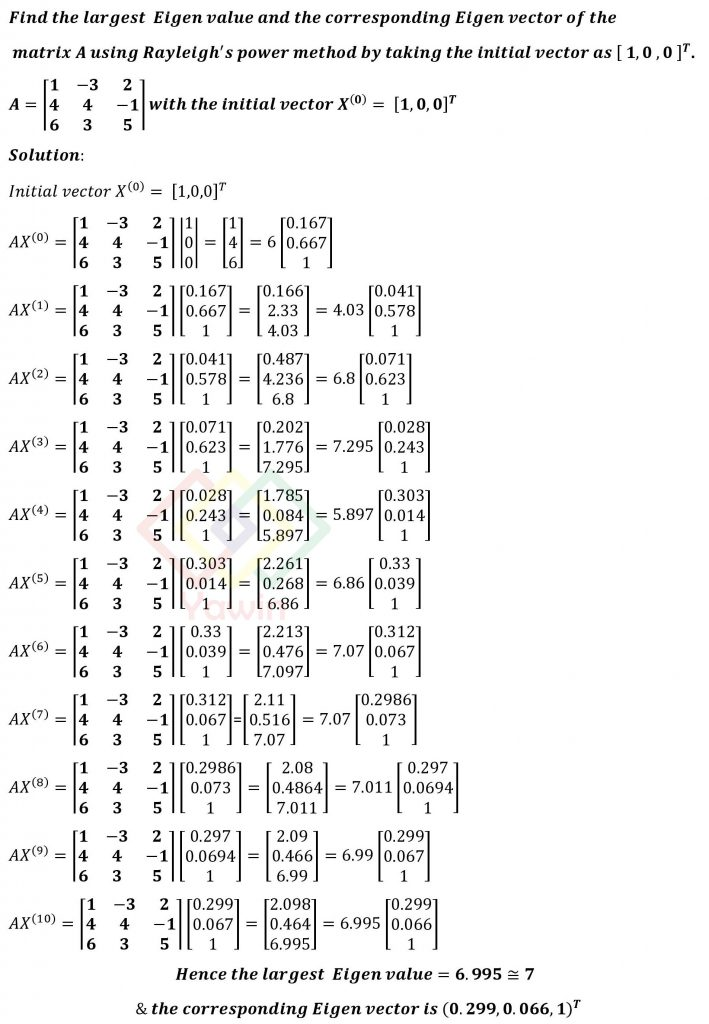Find the largest Eigen value and the corresponding Eigen vector of the matrix A using Rayleigh’s power method by taking the initial vector as [ 1,0 ,0 ]^T. A = (1 – 3 2 4 4 – 1 6 3 5) with the initial vector X^((0))= [1,0,0]^T

## Problem## Question

Find the largest Eigen value and the corresponding Eigen vector of the matrix A using Rayleigh’s power method by taking the initial vector as [ 1,0 ,0 ]^T. A = (1 – 3 2 4 4 – 1 6 3 5) with the initial vector X^((0))= [1,0,0]^T

## Topic

Rayleigh’s Power Method